Get inspired by the success stories of our students in IIT JAM MS, ISI  MStat, CMI MSc DS.  Learn More

# ISI MStat PSB 2007 Problem 3 | Application of L'hospital RuleThis is a very beautiful sample problem from ISI MStat PSB 2007 Problem 3 based on use of L'hospital Rule . Let's give it a try !!

## Problem- ISI MStat PSB 2007 Problem 3

Let f be a function such thatand f has derivatives of all order. Show that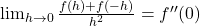where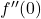is the second derivative of f at 0.

### Prerequisites

Differentiability

Continuity

L'hospital rule

## Solution :

Let L=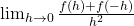it's aform as f(0)=0 .

So , here we can use L'hospital rule as f is differentiable .

We get L=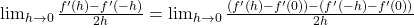=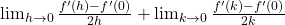, taking -h=k .

==. Hence done!

## Food For Thought

Letbe a continuous function such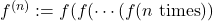and assume that there exists a positive integer m such that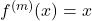for all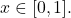Prove that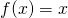for all## Subscribe to Cheenta at Youtube

This is a very beautiful sample problem from ISI MStat PSB 2007 Problem 3 based on use of L'hospital Rule . Let's give it a try !!

## Problem- ISI MStat PSB 2007 Problem 3

Let f be a function such thatand f has derivatives of all order. Show thatwhereis the second derivative of f at 0.

### Prerequisites

Differentiability

Continuity

L'hospital rule

## Solution :

Let L=it's aform as f(0)=0 .

So , here we can use L'hospital rule as f is differentiable .

We get L==, taking -h=k .

==. Hence done!

## Food For Thought

Letbe a continuous function suchand assume that there exists a positive integer m such thatfor allProve thatfor all## Subscribe to Cheenta at Youtube

This site uses Akismet to reduce spam. Learn how your comment data is processed.

### Knowledge Partner